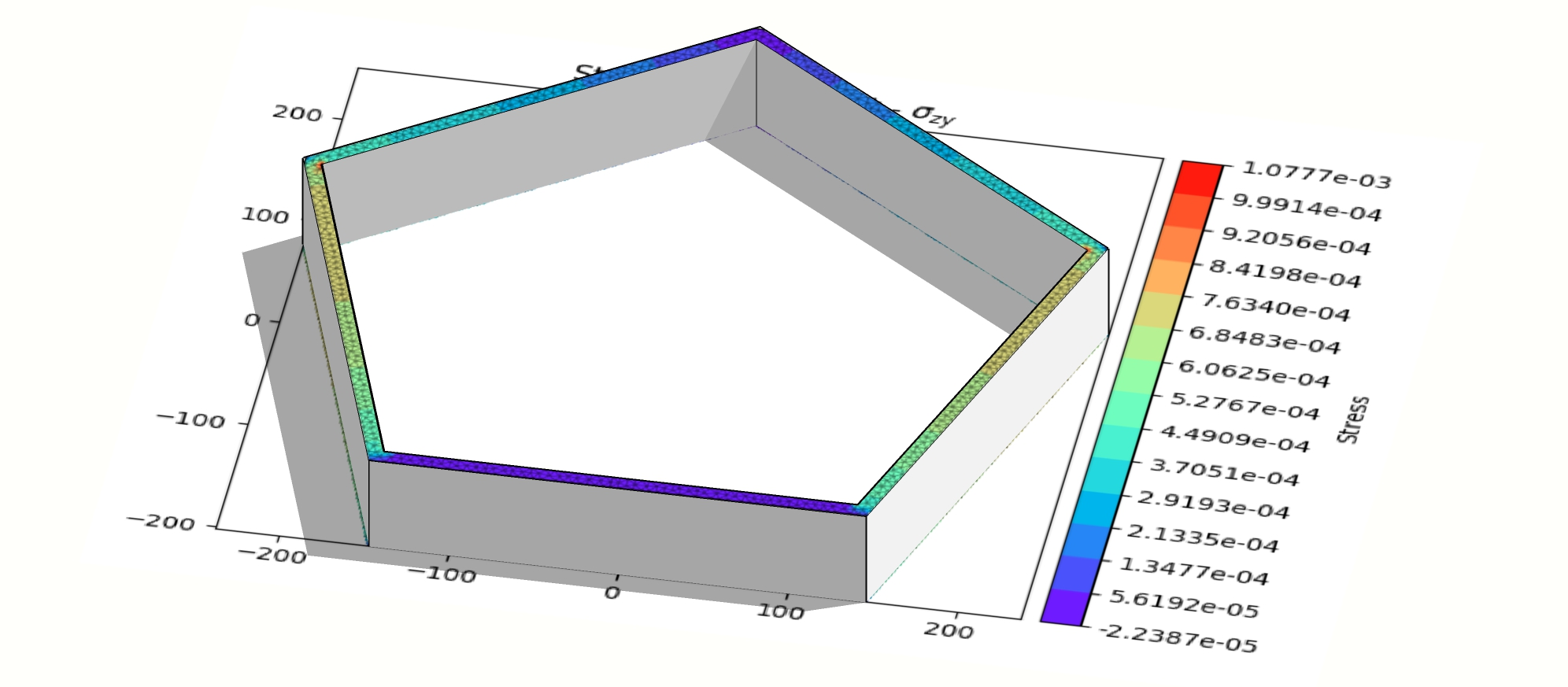Tower Engineering

# An alternative way of getting cross-section properties of polygonal, thin-walled membersAndrzej Baczkowicz
Oct 31, 2023
7The shear effects in finite element analysis of stocky frame structures significantly contributes to the overall structure deformation, but the determination of cross-section shear properties might become a challenging process that takes hours of computation.

In contrast to the common cross-sections, such as rectangular or circular hollow sections, where analytical formulas can be used, obtaining properties of non-standard or build-up sections requires time-consuming section meshing and running finite element analysis.

Recently I ran the analysis of a 20 meter high tapered shape monopole built from 5-sided polygonal, thin-walled elements. Having divided the pole structure into finite elements, I've obtained 101 members of individual geometrical properties.

As a result, I've been pushed to run the finite element method at 101 different sections, which took 201.89s (3min 22s) of computation time. Scaling this to the much bigger task of analyzing 1000 similar structures in one turn led me to the conclusion that such a process can take 56 hours. Such a long procedure didn't satisfy my expectations.

Hoping to reduce the computation time, I've decided to search for an analytical formula defining the shear area from diameter and thickness based on the large number of data obtained from a single-time-only finite element analysis process of finding the 5-sided polygon-shaped section properties from 1324 geometrical variations.

Then, I was able to run a multiple linear regression analysis in the Python code and find the formula for the shear area property. The goal of regression analysis is to fit the variable data into the result's model and return Y = A + B1X1 + B2X2 + B3X3 ... formula governed by the lowest model's error value, where Y represents the expected result, A is the intercept value and B1...Bn state for coefficients applied to the explanatory variables.

A regression analysis solution of 5-sided polygon is as follows:

As = (0.444526162934341 - 0.000002906631453 * d + 0.000389920125454 * t ) * A

where:
d
- diameter of circumcircle of an outer 5-sided polygon
t
- thickness of element
A
- cross-section area

Computing the values again with the formula proved that there is a negligible difference in the shear area obtained from FEA and analytical calculations and the maximum deviation from the original result is +0.3823%.

In the same process the computation time had reduced from 2644.22s to 0.0453s per 1324 cross-sections, which makes the 56 hours potentially spent on the analysis of 1000 structures diminish to 3.46s only.